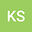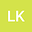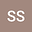Finite Element Analysis of Time-Fractional Integro-differential Equation of Kirchhoff type for Non-homogeneous Materials
•••• Lalit Kumar,
• Sivaji Ganesh Sista
Indian Institute of Technology Delhi

Author ProfileLalit Kumar
Indian Institute of Technology Bombay Department of Mathematics
Author ProfileSivaji Ganesh Sista
Indian Institute of Technology Bombay Department of Mathematics
Author Profile## Abstract

In this paper, we study a time-fractional initial-boundary value problem of Kirchhoff type involving memory term for non-homogeneous materials ( P α ). As a consequence of energy argument, we derive L ∞ ( 0 , T ; H 0 1 ( Ω ) ) bound as well as L 2 ( 0 , T ; H 2 ( Ω ) ) bound on the solution of the problem ( P α ) by defining two new discrete Laplacian operators. Using these a priori bounds, existence and uniqueness of the weak solution to the considered problem is established. Further, we study semi discrete formulation of the problem ( P α ) by discretizing the space domain using a conforming FEM and keeping the time variable continuous. The semi discrete error analysis is carried out by modifying the standard Ritz-Volterra projection operator in such a way that it reduces the complexities arising from the Kichhoff type nonlinearity. Finally, we develop a new linearized L1 Galerkin FEM to obtain numerical solution of the problem ( P α ) with a convergence rate of O ( h + k 2 − α ) , where α (0 1) is the fractional derivative exponent, h and k are the discretization parameters in the space and time directions respectively. This convergence rate is improved to second order in the time direction by proposing a novel linearized L2-1 σ Galerkin FEM. We conduct a numerical experiment to validate our theoretical claims.